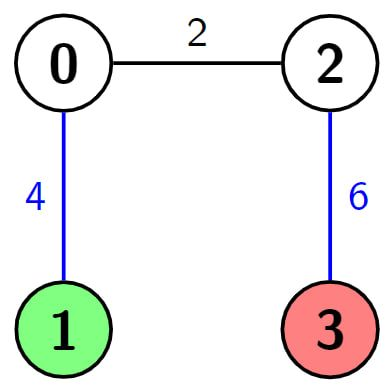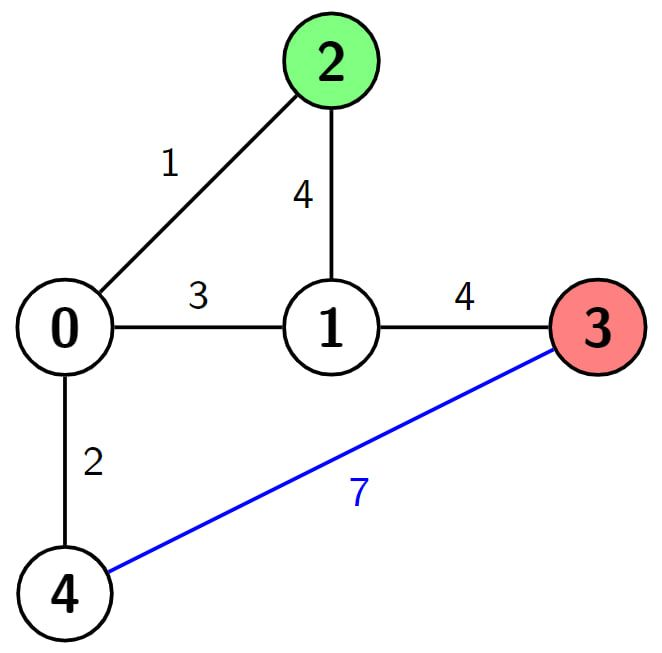# 2714. Find Shortest Path with K Hops

## Description

You are given a positive integer n which is the number of nodes of a 0-indexed undirected weighted connected graph and a 0-indexed 2D array edges where edges[i] = [ui, vi, wi] indicates that there is an edge between nodes ui and vi with weight wi.

You are also given two nodes s and d, and a positive integer k, your task is to find the shortest path from s to d, but you can hop over at most k edges. In other words, make the weight of at most k edges 0 and then find the shortest path from s to d.

Return the length of the shortest path from s to d with the given condition.

Example 1:

Input: n = 4, edges = [[0,1,4],[0,2,2],[2,3,6]], s = 1, d = 3, k = 2
Output: 2
Explanation: In this example there is only one path from node 1 (the green node) to node 3 (the red node), which is (1->0->2->3) and the length of it is 4 + 2 + 6 = 12. Now we can make weight of two edges 0, we make weight of the blue edges 0, then we have 0 + 2 + 0 = 2. It can be shown that 2 is the minimum length of a path we can achieve with the given condition.Example 2:

Input: n = 7, edges = [[3,1,9],[3,2,4],[4,0,9],[0,5,6],[3,6,2],[6,0,4],[1,2,4]], s = 4, d = 1, k = 2
Output: 6
Explanation: In this example there are 2 paths from node 4 (the green node) to node 1 (the red node), which are (4->0->6->3->2->1) and (4->0->6->3->1). The first one has the length 9 + 4 + 2 + 4 + 4 = 23, and the second one has the length 9 + 4 + 2 + 9 = 24. Now if we make weight of the blue edges 0, we get the shortest path with the length 0 + 4 + 2 + 0 = 6. It can be shown that 6 is the minimum length of a path we can achieve with the given condition.Example 3:

Input: n = 5, edges = [[0,4,2],[0,1,3],[0,2,1],[2,1,4],[1,3,4],[3,4,7]], s = 2, d = 3, k = 1
Output: 3
Explanation: In this example there are 4 paths from node 2 (the green node) to node 3 (the red node), which are (2->1->3), (2->0->1->3), (2->1->0->4->3) and (2->0->4->3). The first two have the length 4 + 4 = 1 + 3 + 4 = 8, the third one has the length 4 + 3 + 2 + 7 = 16 and the last one has the length 1 + 2 + 7 = 10. Now if we make weight of the blue edge 0, we get the shortest path with the length 1 + 2 + 0 = 3. It can be shown that 3 is the minimum length of a path we can achieve with the given condition.Constraints:

• 2 <= n <= 500
• n - 1 <= edges.length <= min(104, n * (n - 1) / 2)
• edges[i].length = 3
• 0 <= edges[i], edges[i] <= n - 1
• 1 <= edges[i] <= 106
• 0 <= s, d, k <= n - 1
• s != d
• The input is generated such that the graph is connected and has no repeated edges or self-loops

## Solutions

• class Solution {
public int shortestPathWithHops(int n, int[][] edges, int s, int d, int k) {
List<int[]>[] g = new List[n];
Arrays.setAll(g, i -> new ArrayList<>());
for (int[] e : edges) {
int u = e, v = e, w = e;
}
PriorityQueue<int[]> pq = new PriorityQueue<>((a, b) -> a - b);
pq.offer(new int[] {0, s, 0});
int[][] dist = new int[n][k + 1];
final int inf = 1 << 30;
for (int[] e : dist) {
Arrays.fill(e, inf);
}
dist[s] = 0;
while (!pq.isEmpty()) {
int[] p = pq.poll();
int dis = p, u = p, t = p;
for (int[] e : g[u]) {
int v = e, w = e;
if (t + 1 <= k && dist[v][t + 1] > dis) {
dist[v][t + 1] = dis;
pq.offer(new int[] {dis, v, t + 1});
}
if (dist[v][t] > dis + w) {
dist[v][t] = dis + w;
pq.offer(new int[] {dis + w, v, t});
}
}
}
int ans = inf;
for (int i = 0; i <= k; ++i) {
ans = Math.min(ans, dist[d][i]);
}
return ans;
}
}

• class Solution {
public:
int shortestPathWithHops(int n, vector<vector<int>>& edges, int s, int d, int k) {
vector<pair<int, int>> g[n];
for (auto& e : edges) {
int u = e, v = e, w = e;
g[u].emplace_back(v, w);
g[v].emplace_back(u, w);
}
priority_queue<tuple<int, int, int>, vector<tuple<int, int, int>>, greater<tuple<int, int, int>>> pq;
pq.emplace(0, s, 0);
int dist[n][k + 1];
memset(dist, 0x3f, sizeof(dist));
dist[s] = 0;
while (!pq.empty()) {
auto [dis, u, t] = pq.top();
pq.pop();
for (auto [v, w] : g[u]) {
if (t + 1 <= k && dist[v][t + 1] > dis) {
dist[v][t + 1] = dis;
pq.emplace(dis, v, t + 1);
}
if (dist[v][t] > dis + w) {
dist[v][t] = dis + w;
pq.emplace(dis + w, v, t);
}
}
}
return *min_element(dist[d], dist[d] + k + 1);
}
};

• class Solution:
def shortestPathWithHops(
self, n: int, edges: List[List[int]], s: int, d: int, k: int
) -> int:
g = [[] for _ in range(n)]
for u, v, w in edges:
g[u].append((v, w))
g[v].append((u, w))
dist = [[inf] * (k + 1) for _ in range(n)]
dist[s] = 0
pq = [(0, s, 0)]
while pq:
dis, u, t = heappop(pq)
for v, w in g[u]:
if t + 1 <= k and dist[v][t + 1] > dis:
dist[v][t + 1] = dis
heappush(pq, (dis, v, t + 1))
if dist[v][t] > dis + w:
dist[v][t] = dis + w
heappush(pq, (dis + w, v, t))
return int(min(dist[d]))


• func shortestPathWithHops(n int, edges [][]int, s int, d int, k int) int {
g := make([][]int, n)
for _, e := range edges {
u, v, w := e, e, e
g[u] = append(g[u], int{v, w})
g[v] = append(g[v], int{u, w})
}
pq := hp{ {0, s, 0} }
dist := make([][]int, n)
for i := range dist {
dist[i] = make([]int, k+1)
for j := range dist[i] {
dist[i][j] = math.MaxInt32
}
}
dist[s] = 0
for len(pq) > 0 {
p := heap.Pop(&pq).(tuple)
dis, u, t := p.dis, p.u, p.t
for _, e := range g[u] {
v, w := e, e
if t+1 <= k && dist[v][t+1] > dis {
dist[v][t+1] = dis
heap.Push(&pq, tuple{dis, v, t + 1})
}
if dist[v][t] > dis+w {
dist[v][t] = dis + w
heap.Push(&pq, tuple{dis + w, v, t})
}
}
}
ans := math.MaxInt32
for i := 0; i <= k; i++ {
ans = min(ans, dist[d][i])
}
return ans
}

func min(a, b int) int {
if a < b {
return a
}
return b
}

type tuple struct{ dis, u, t int }
type hp []tuple

func (h hp) Len() int           { return len(h) }
func (h hp) Less(i, j int) bool { return h[i].dis < h[j].dis }
func (h hp) Swap(i, j int)      { h[i], h[j] = h[j], h[i] }
func (h *hp) Push(v any)        { *h = append(*h, v.(tuple)) }
func (h *hp) Pop() any          { a := *h; v := a[len(a)-1]; *h = a[:len(a)-1]; return v }